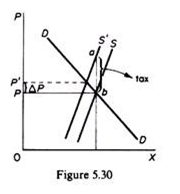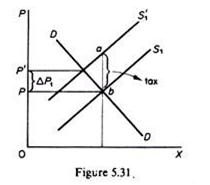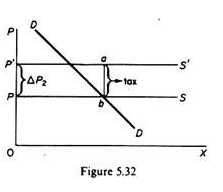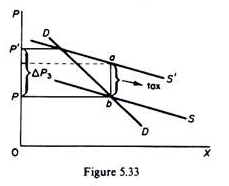# Imposition of Tax by the Government: Lump-Sum, Profit and Specific Tax

We will examine the effects of the imposition by the government of a lump-sum tax, a profits tax, and a specific tax, that is, a tax per unit of output.

#### Imposition of a lump-sum tax (per period):

In the short run the lump-sum tax will not affect the MC cost curve and the firm will continue to produce the same output as before the imposition of the tax.

However, if the firm was earning just normal profits prior to the tax, it will not be covering its ATC at the going market price and will close down in the long run. Thus in the long run the market-supply curve will shift to the left as firms leave the industry; the output will be lower and the price higher as compared with the pre-tax equilibrium.

#### Imposition of a profits tax:

This tax takes the form of a percentage on the net profit of the firm. The effects of a profits tax are the same with those of a lump-sum tax. The profits tax, while reducing the profits (by adding to the cash expenses of the firm), will not affect its MC. Hence in the short run the equilibrium of the firm and the industry will not change. However, in the long run, exit of firms will be inevitable if in the pre-tax period firms were earning just normal profits. In the long run the supply in the market would shift to the left and a new equilibrium would be reached with a higher price, a lower quantity produced and a smaller number of firms.

#### Imposition of a specific sales tax:

This takes the form of a given amount of money (e.g. 5p) per unit of output produced. Such a tax clearly affects the MC of the firm. The MC curve, which is also the supply curve of the firm, will shift upwards to the left, and the amount produced at the going price will be reduced. The market-supply curve will shift upwards to the left and price will rise. The im­portant question here is by how much will the price increase: will the increase in P be smaller, equal, or greater than the specific tax? This is an important question because it relates to who is going to bear the specific sales tax: the consumer-buyer, the firm, or both?

The answer to this question is that the burden of the specific tax that will be borne by the consumer (buyer) depends on the price elasticity of supply, given the market demand. In general, the most elastic the market supply the higher the proportion of the tax that the consumer will bear and the less the burden of the firm from the specific tax.

So long as the market supply has a positive slope the specific tax will be paid partly by the buyer and partly by the firm. The burden to the firm will be smaller the greater the elasticity of supply. In other words, the firms will be able to pass on to the consumer more of the specific tax, the more elastic the market supply.

This is illustrated in figures 5.30 and 5.31. The demand curve is identical in both figures and the initial (pre-tax) price is the same, but the supply in figure 5.31 is more elastic. Imposition of a specific tax equal to ab raises the price by ΔP in figure 5.30 and by ΔP, in figure 5.31. Clearly ΔP1 > ΔP, that is, the tax burden to the consumer is greater in the case of a more elastic supply curve (given the market demand).In the limiting case of a market-supply curve with infinite elasticity the increase in price is equal to the specific tax and the whole tax burden is borne by the consumer. In figure 5.32 the demand is the same as in figures 5.30 and 5.31, but the supply is parallel to the horizontal axis, showing infinite price elasticity. The imposition of a specific tax equal to ab (same as before) leads to an equal increase in the price ∆P2 = ab.

If the supply curve has a negative slope (figure 5.33) the imposition of a specific tax results in an increase in the price which is greater than the tax. In figure 5.33 the demand is identical as in the above-examined cases but the supply is negatively sloping (with its slope smaller than the slope of the DD curve). Under these conditions a specific tax of ab leads to an increase in the market price equal to ∆P3, which is obviously larger than the unit tax.The analysis of the predictions of the perfect competition model when the environ­ment changes is important. Such analysis will be attempted for other models of the behaviour of the firm. Comparison of the predictions of various models usually shows how to test the different theories and choose between them.